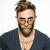# These Difficult Equations Will Make You Smarter

## Test your math skills with this test. Only 15 questions and it's yours.Created by Terry Stein
On Jan 26, 2022
1 / 15

8 + 8 ÷ 8 + 8 x 8 – 8 = ?

2 / 15

1 + 1 × ¼ + 1 − 1 = ?

3 / 15

20 ÷ 4 x 10 ÷ 2 = ?

4 / 15

2 + 1 ÷ 1 + 1 x 2 = ?

5 / 15

16 ÷ ¼ + 4 = ?

6 / 15

20 ÷ 4 x 20 ÷ 5 = ?

7 / 15

20 – 20 ÷ ¼ + 1 = ?

8 / 15

10 x 1 + 10 ÷ 1 = ?

9 / 15

6 + 6 x 6 – 6 = ?

10 / 15

1600/40 = ?

11 / 15

15 ÷ 5 + 15 ÷ 5 = ?

12 / 15

16 ÷ 2 (8 – 6) = ?

13 / 15

7 + 1 x 1 + 8 ÷ 2 = ?

14 / 15

5 + 1 x 0 + 8 ÷ 2 = ?

15 / 15

And finally, which is divisible by 7 without a remainder?

15
Questions left

### How much did you like this quiz?

Calculating results